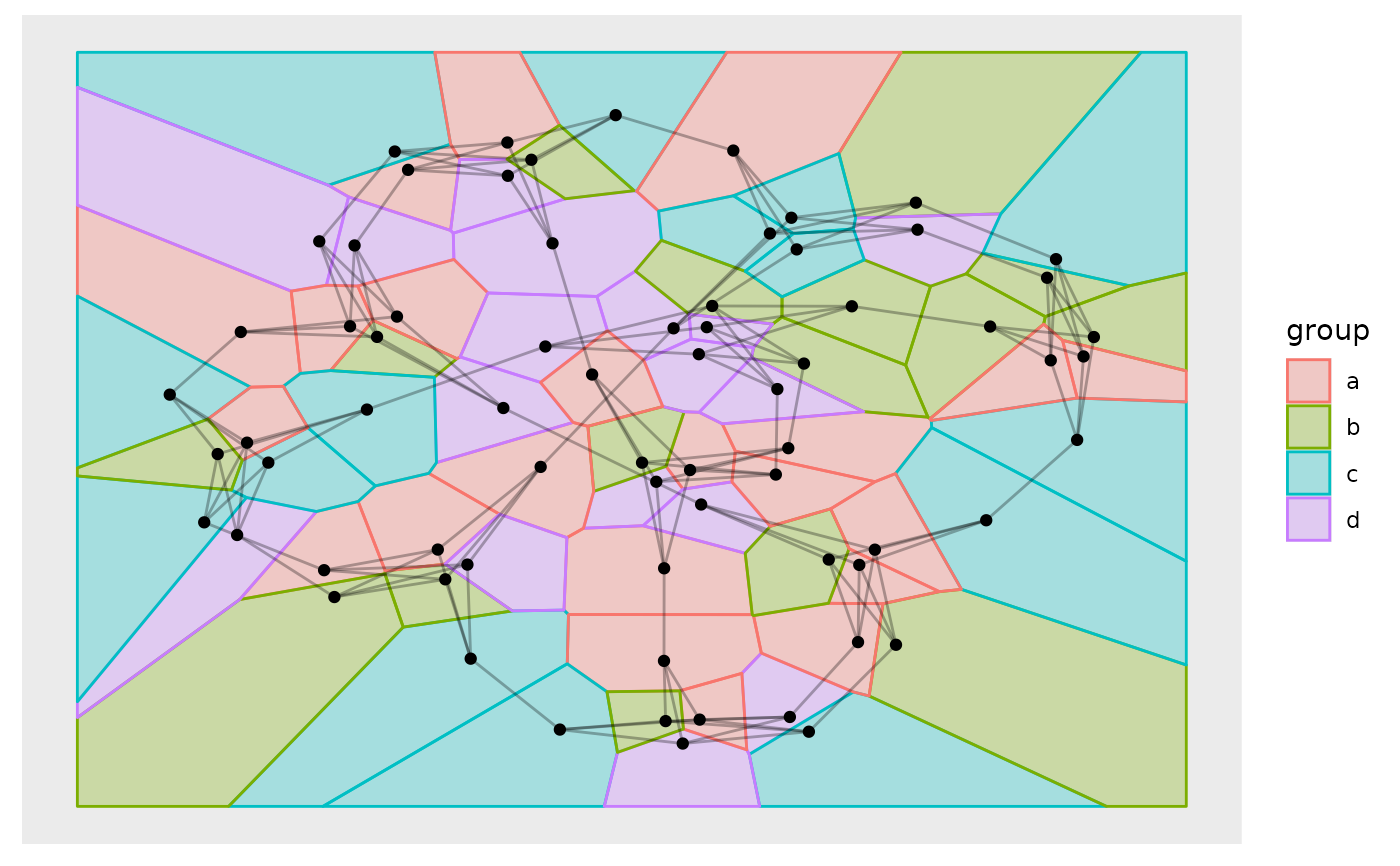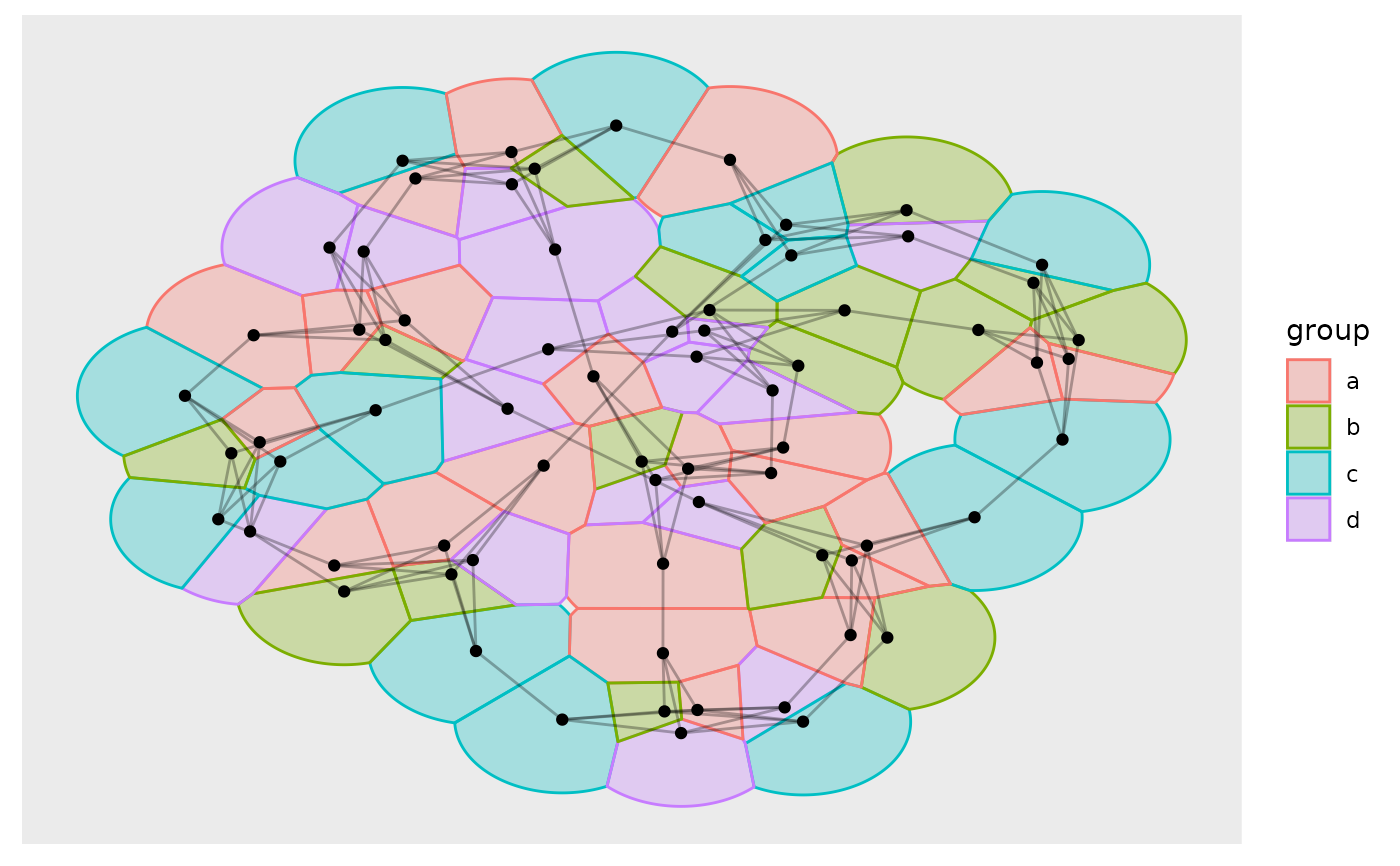This geom is equivalent in functionality to ggforce::geom_voronoi_tile() and allows for plotting of nodes as tiles from a voronoi tesselation. As with ggforce::geom_voronoi_tile() it is possible to restrict the size of the tile to a fixed radius, as well as round corners and expand/contract the tile.

geom_node_voronoi(
mapping = NULL,
data = NULL,
position = "identity",
show.legend = NA,
bound = NULL,
eps = 1e-09,
normalize = FALSE,
asp.ratio = 1,
expand = 0,
...
)

## Arguments

mapping

Set of aesthetic mappings created by ggplot2::aes() or ggplot2::aes_(). By default x and y are mapped to x and y in the node data and group set to -1.

data

The data to be displayed in this layer. There are three options:

If NULL, the default, the data is inherited from the plot data as specified in the call to ggplot().

A data.frame, or other object, will override the plot data. All objects will be fortified to produce a data frame. See fortify() for which variables will be created.

A function will be called with a single argument, the plot data. The return value must be a data.frame, and will be used as the layer data. A function can be created from a formula (e.g. ~ head(.x, 10)).

position

Position adjustment, either as a string, or the result of a call to a position adjustment function.

show.legend

logical. Should this layer be included in the legends? NA, the default, includes if any aesthetics are mapped. FALSE never includes, and TRUE always includes. It can also be a named logical vector to finely select the aesthetics to display.

bound

The bounding rectangle for the tesselation or a custom polygon to clip the tesselation to. Defaults to NULL which creates a rectangle expanded 10\ vector giving the bounds in the following order: xmin, xmax, ymin, ymax. If supplied as a polygon it should either be a 2-column matrix or a data.frame containing an x and y column.

eps

A value of epsilon used in testing whether a quantity is zero, mainly in the context of whether points are collinear. If anomalous errors arise, it is possible that these may averted by adjusting the value of eps upward or downward.

The maximum distance a tile can extend from the point of origin. Will in effect clip each tile to a circle centered at the point with the given radius. If normalize = TRUE the radius will be given relative to the normalized values

normalize

Should coordinates be normalized prior to calculations. If x and y are in wildly different ranges it can lead to tesselation and triangulation that seems off when plotted without ggplot2::coord_fixed(). Normalization of coordinates solves this. The coordinates are transformed back after calculations.

asp.ratio

If normalize = TRUE the x values will be multiplied by this amount after normalization.

expand

A numeric or unit vector of length one, specifying the expansion amount. Negative values will result in contraction instead. If the value is given as a numeric it will be understood as a proportion of the plot area width.

As expand but specifying the corner radius.

...

Other arguments passed on to layer(). These are often aesthetics, used to set an aesthetic to a fixed value, like colour = "red" or size = 3. They may also be parameters to the paired geom/stat.

## Aesthetics

geom_node_voronoi understand the following aesthetics. Bold aesthetics are automatically set, but can be overridden.

• x

• y

• alpha

• colour

• fill

• shape

• size

• stroke

• filter

Other geom_node_*: geom_node_arc_bar(), geom_node_circle(), geom_node_point(), geom_node_range(), geom_node_text(), geom_node_tile()

## Author

Thomas Lin Pedersen

## Examples

require(tidygraph)
gr <- create_notable('meredith') %>%
mutate(group = sample(letters[1:4], n(), TRUE))

ggraph(gr) +
geom_node_voronoi(aes(fill = group, colour = group), alpha = 0.3) +
#> Using stress as default layout#> Using stress as default layout